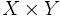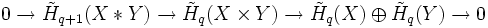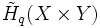# Exact sequence for join and product

## Statement

Let$X$ and$Y$ be topological spaces. Denote by$X * Y$ the join and by$X \times Y$ the product. The long exact sequence of reduced homology obtained using Mayer-Vietoris then splits into short exact sequences of the form:$0 \to \tilde{H}_{q+1}(X * Y) \to \tilde{H}_q(X \times Y) \to \tilde{H}_q(X) \oplus \tilde{H}_q(Y) \to 0$

Moreover, this short exact sequence splits so we get:$\tilde{H}_q(X \times Y) \cong \tilde{H}_{q+1}(X * Y) \oplus \tilde{H}_q(X) \oplus \tilde{H}_q(Y)$

Note that the above is not true for unreduced homology at$q = 0$.

## Applications

In the case when either$X$ or$Y$ is a sphere, the homology of$X \times Y$ can be computed in terms of the homology of$X$. This is because taking a join with a sphere, is equivalent to an iterated suspension, and the homology of an iterated suspension is simply obtained by displacing the homology of the original space.

In symbols:$\tilde{H}_q(X \times S^m) = \tilde{H}_{q-m}(X) \oplus \tilde{H}_q(X) + \tilde{H}_q(S^m)$

The utility of this exact sequence in computing the homology of the product breaks down when we do not understand the homologies of the join. In this case, we use a more advanced tool such as the Kunneth formula.

## Proof

We can view$X * Y$ as the double mapping cylinder for the coordinate projections from$X \times Y$ to$X$ and to$Y$ and then apply the exact sequence for double mapping cylinder. This is a long exact sequence. To show that it separates into several short exact sequence, and that each one splits, it suffices to construct a section of the map from$\tilde{H}_q(X) \oplus \tilde{H}_q(Y)$ to$\tilde{H}_q(X \times Y)$. For$q \ge 1$, this section can be constructed geometrically, and for$q = 0$ it can be constructed explicitly in terms of the description of reduced homology.